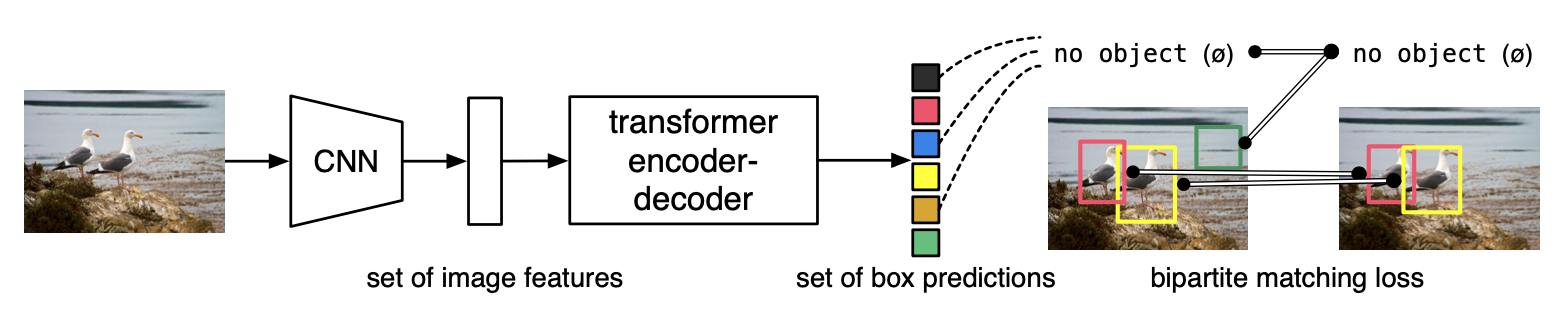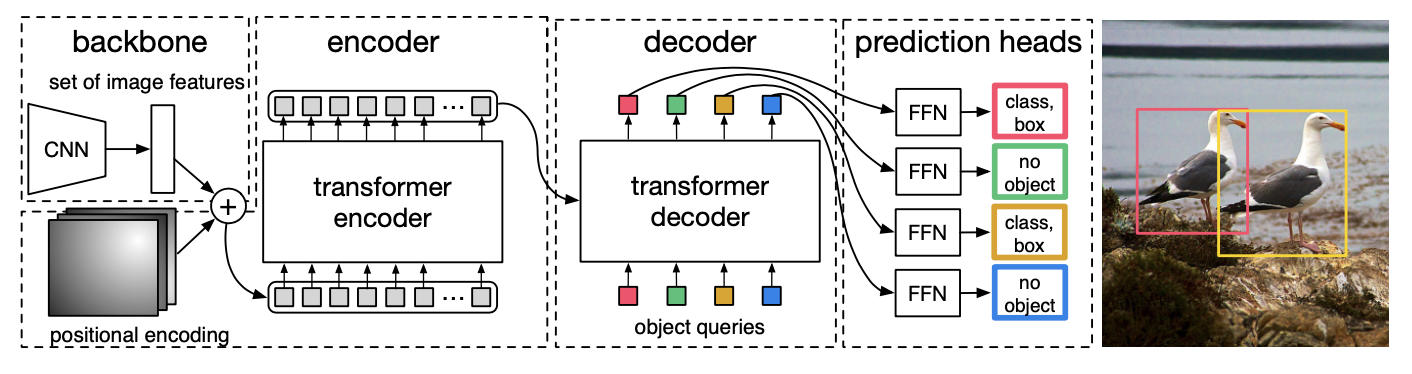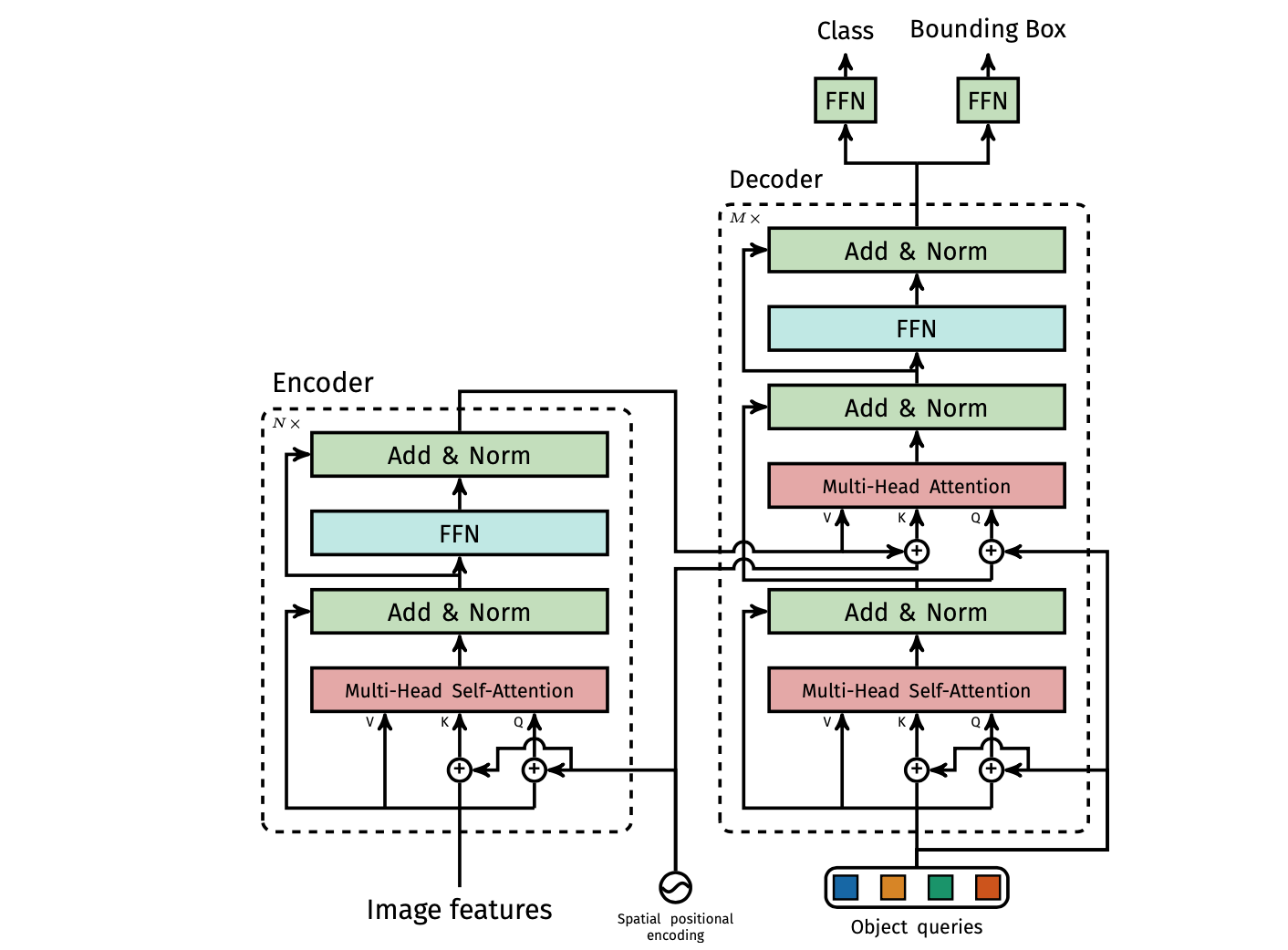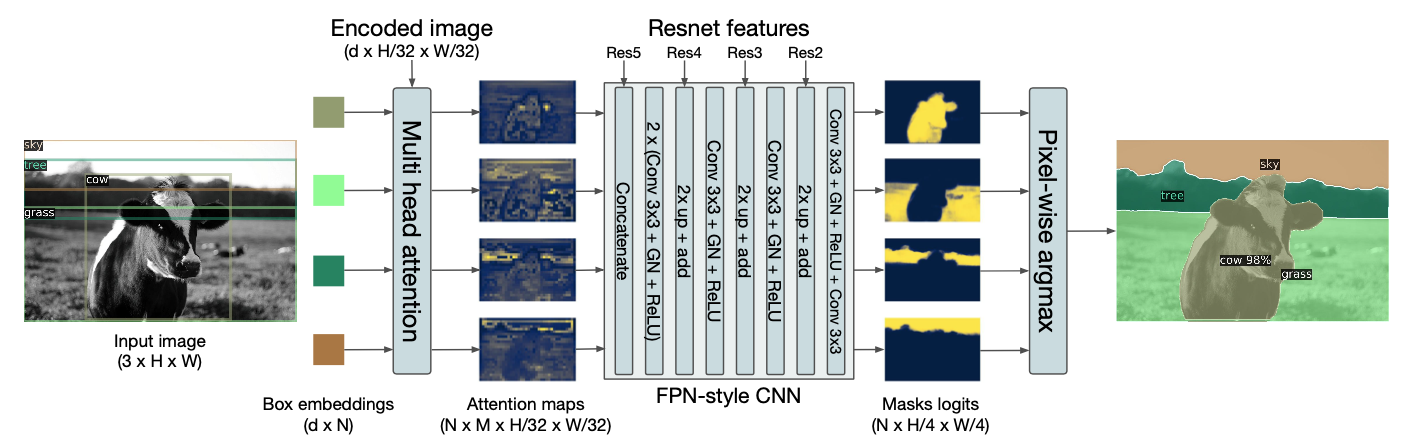# Introduction

Before reading this article, you may have a look at my previous article “Transformer? Attention!” in which I explain “Attention mechanism” and “The Transformer”. Early in 2020, Facebook AI proposed a new way to use the Transformer in object detection task in paper “End-to-End Object Detection with Transformers” in which they present a new method that view object detection as a direct set prediction problem. For now, it only has its basic version that means that it still has quite a lot of potential and can be optimized. However, it has already significantly outperformed competitive baselines for accuracy and run-time.

There are four special characteristics for this model:

1. DETR predicts all objects at once and is trained end-to-end.
2. DETR does not have multiple hand-designed components that encode prior knowledge, like sliding windows, spatial anchors, non-maximal suppression, large set of proposals, or window centers.
3. DETR does not require any customized layers, and thus can be reproduced easily in any framework that conatins standard CNN and transformer classes.
4. DETR can be easily generalized to produce panoptic segmentation in a unified manner.

Training code and pretrained models are available at “End-to-End Object Detection with Transformers”.

# Model ArchitectureThe overall DETR architecture is surprisingly simple. It contains three main components.

1. A CNN backbone to extract a compact feature representation.
2. An encoder-decoder transformer.
3. A simple feed forward network(FFN) that makes the final detection prediction.

## Backbone

Starting from the initial image $x_{img}\in R^{3\times H_0\times W_0}$(with 3 color channels) and All imput image are batched together, applying 0-padding adequately to ensure they all have the same dimensions ($H_0, W_0$) as the largest image of the batch. The conventional CNN backbone generats a lower-resolution activation map $f\in R^{C\times H\times W}$ as a set of image features. The dimensions of this feature map are $C = 2048$ and $H,W = \frac{H_0}{frac}, \frac{W_0}{frac}$ where frac is 32 for DETR and 16 for DETR DC5.

## Transformer encoder

Each encoder layer has a standard architecture ( “Attention is all you need”)and consist of a multi-head self-attention module and a feed forward network (FFN). The encoder follows the following steps:

1. Use a $1\times1$ convolutional kernal to reduces the channel dimension of the high-level activation map $f$ of dimension $R^{C\times H\times W}$ to a new feature map $z_0$ of dimension $R^{d\times H\times W}$ where $d$ is smaller than $C$.
2. Collapse $z_0$ into one dimension, resulting in a $d\times HW$ feature map which is the input sequence for the encoder.
3. The transformer architecture is permutation-invariant, we supplement it with fixed positional encodings that are added to the input of each attention layer(how? in this case)..

## Transformer decoder

The decoder follows the standard architecture of the transformer too, transforming $N$ embeddings of size $d$ using multi-headed self- and encoder-decoder attention mechanism. To be noted that this model decodes the $N$ objects in parallel at each decoder layer(not autoregressive). Since the decoder is permutation-invariant, the N input embeddings must be different to produce different results. These input embeddings are learnt positional encodings that we refer to as Object Queries. These queries are added to the input of each attention layer similarly to the encoder.

It follows the following steps:

1. The $N$ object queries are transformed into an output embedding by the decoder.
2. The output embeddings are independent decoded into box coordinates and class labels by a feed forward network, resulting N final predictions.

Using self- and encoder-decoder attention over these embeddings, the model globally reasons about all objects together using pair-wise relations between them, while being able to use the whole image as context.

## Prediction feed-forward networks(FFNs)

$$FFN(x) = RELU(xW_1 + b_1)W_2 + b_2$$

The final prediction is computed a 3-layer perceptron with RELU activation function and hidden dimension $d$, and a linear projection layer. Like YOLO, The FFN predicts the normalized center coordinates, height and weidth of the box w.r.t. the input image. It predicts also the class label using a softmax function. An additional special class label $\varnothing$ is introduced and is used to represent that no object is detected within a slot (The “background” class) as we predict a fixed-size set of N bounding box, where N is usually much larger than the actual number of objects of interest in an image.

## Auxiliary decoding losses

It is helpful to use auxiliary losses as  “Character-Level Language Modeling with Deeper Self-Attention” in decoder during training, espacially to help the model output the correct number of object of each class. The idea is to add prediction FFNs and Hungarian loss after each decoder layer. All predictions FFNs share their parameters. An additional shared layer-norm is used to normalize the input to the prediction FFNs rom different decoder layer.

## Detailed model architecture### Transformer architecture# Set Prediction and Loss function

As we mentioned at the beginning, this model views object detection as a direct set prediction problem.The basic set prediction task is mutilabel classification and is does not apply to problem such as detection where there is an underlying structure between elements(i.e., near-identical boxes which need post-processing like NMS to eliminate duplicates). So we designed a loss based on the Hungarian algorithm to find a biopartite matching between ground-truth and prediction. In this way, it enforces permutation-invariance, and guarantees that each target element has a unique match by making the model to learning the underlying structure between elements and makes the pipeline post-processing free. To ensure the performance, we use also parallel decoding for the transformer.

The key element for training prossess is the loss function. DETR infers a fixed-size set of N predictions, in a single pass through the decoder, where N is set to be significantly larger thatn the typical number of object in an image and we need to score predicted objects (class, position, size) with respect to the ground truth. So the loss function following two steps:

1. Produce an optimal bipartite matching between predicted and ground truth objects.
2. Optimize object-specific (bounding box) losses.

## Search for the permutation

This part, we will try to find the optimal permutation of $N$ predicted elements $\hat{\sigma} \in \mathcal{G}$. $y$ is the ground truth set of objects, and $\hat{y} = \{\hat{y}_i \}_{i=1}^{N}$.
Given that the size of y is smaller than $N$, it will be padded with $\varnothing$.

$$\hat{\sigma} = \arg min_{\sigma \in \mathcal{G}_N} \sum_{i}^{N}\mathcal{L}_{match}(y_i, \hat{y}_{\sigma(i)}),$$

where $\mathcal{L}_{match}(y_i, \hat{y}_{\sigma(i)})$ is a paire-wise matching cost between ground truth $y_i$ and a prediction with index $\sigma(i)$. This optimal assignment is computed efficiently with the Hungarian algorithm, following prior work (e.g. “End-to-end people detection in crowded scenes”).

### Matching cost

The matching cost takes into account:

1. The class prediction. We define $c_i$ as the target class label of the ith ground truth.
2. The similarity of predicted and ground truth boxes. We define $b_i \in [0 ,1]^4$ as a vector that defines ground truth box center coordinates and its heigt and width relative to the image size.

Each element i of the ground truth set can be seen as a $y_i = (c_i, b_i)$. For the prediction with the permutation $\sigma(i)$ we define probability of class $c_i$ as $\hat{p}_{\sigma(i)}(c_i)$ and the predicted box as $\hat{b}_{\sigma(i)}$. We define :

$$\mathcal{L}_{match}(y_i, \hat{y}_{\sigma(i)}) = \underbrace{-\unicode{x1D7D9}_{\{c_i\neq\varnothing\}}\hat{p}_{\sigma(i)}(c_i)}_{\text{Higher probability of right class}} + \underbrace{\unicode{x1D7D9}_{\{c_i\neq\varnothing\}}\mathcal{L}_{box}(b_i, \hat{b}_{\sigma(i)})}_{\text{Closer bounding box}}$$
By using the permutation, we assume that we are finding one-to-one matching for direct set prediction without duplicates.
In the matching cost we use probabilities $\hat{p}_{\hat{\sigma}(i)}(c_i)$ instead of log-probabilities. This makes the class prediction term commensurable to $\mathcal{L}_{box}(.,.)$.

## Loss function

After finding our optimal permutation $\hat{\sigma}$ in the last procedure, we compute the loss function in this step. The loss function is defined as a linear combination of a negative log-likelihood for class prediction and a box loss defined later:

$$\mathcal{L}_{Hungarian}(y, \hat{y}) = \sum_{i=1}^{N}\left[ -log\hat{p}_{\hat{\sigma}(i)}(c_i) + \unicode{x1D7D9}_{\{c_i\neq\varnothing\}}\mathcal{L}_{box}(b_i, \hat{b}_{\hat{\sigma}(i)}) \right],$$

To be noted that during the procedure of finding the permutation, we do not take the class label probability for $c_i = \varnothing$ into account, while for this loss function, we take it into account. In practice, we down-weight the log-probability term when $c_i = \varnothing$ by a factor 10 to account for class imbalance.

## Bounding box loss

To mitigate the scales problem of $l_1$ loss which induce the inconsistency between big object and little object, a linear combination of the &l_1& loss and the generalized $IoU$ loss is used.

$$L_{box}(b_i, \hat{b}_{\sigma(i)}) = \lambda_{iou}L_{iou}(b_i, \hat{b}_{\sigma(i)}) + \lambda_{L1}||b_i - \hat{b}_{\sigma(i)}||_1$$

where $\lambda_{iou}$, $\lambda_{L1} \in \mathcal{R}$ are hyperparameters. These two losses are normalized by the number of objects inside the batch.

# DETR for panoptic segmentation.

DETR can be naturally extend by adding a mask head on top of the decoder outputs for panoptic segmentation. This head can be used to produce panoptic segmentation by treating stuff and thing classes in a unified way.To DETR panoptic segmentation has 4 parts:

1. We train DETR to predict boxes around both $stuff$ and $things$ classes on COCO, using the same recipe. (Hungarian matching is computed using distance between boxes, so predicting boexs is required for the training to be possible)
2. A mask head predicts a binary mask for each of the predicted boxes. It takes as input the output of the transformer decoder for each object computes mutli-head(with M heads) attention scores of this embedding over the output of the encoder, generating M attention heatmaps per object in a small resolution.
3. An FPN-like architecture is used to make the final prediction and increase the resolution. The final resolution of the masks has stride 4 and each mask is supervised independently using the DICE/F-1 loss and Focal loss.
4. We predict the final panoptic segmentation we simply use an argmax over the mask scores at each pixel, and assign the corresponding categories to the resulting masks.

# Conclusion

DETR is a new design for object detection systems based on Transformer and bipartite matching loss from direct set prediction. It is one of the most popular model in 2020. Without bells and whistles, it achieves comparable results to a well optimized Faster R-CNN baseline on the challenging COCO dataset.
This new design for detectors also comes with new challenges, in particular regarding training, optimization and performances on small objects and it has large potential to be optimized.

# Citation

@article{zhao_DETR2021,
title={DETR: DEtection TRansformer},
url={https://blog.yunfeizhao.com/2021/04/04/DETR/},
journal={https://blog.yunfeizhao.com/},
author={ZHAO, Yunfei}, year={2021}, month={Mar}}